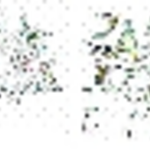Community Profile# Thomas

Last seen: 5 meses ago Active since 2011

Love MATLAB :)
Work with Wireless Sensor Networks, Medical Image processing (MRI,fMRi,DTI), High Performance Computing at UAB
Professional Interests: Parallel Computing, Supercomputing, Wireless sensor networks

#### Statistics

All
••••••••#### Content Feed

View by

Storing values from nested FOR loop (array only saves last run of results)

casi 4 años ago | 6

| accepted

how to find out if a number is even or not
You could perform a modulo division mod(4,2) % Even number ans = 0 mod(5,2) % Odd number ans =1 Answer wi...

casi 4 años ago | 10

| accepted

Counting objects in an image
You could use techniques shown in many MATLAB image processing demos.

casi 5 años ago | 0

how to save a string as a filename?
Maybe something like this for number=1:10 file_name = sprintf('case study_#%d.txt',number); save(file_name,....

más de 5 años ago | 3

| accepted

Distributed and parallel computing without DCS
Hi Chuck, In order to perform distributed computing across multiple computers you need the DCS license. We have DCS running ...

más de 7 años ago | 1

Creating sub-matrices using one matrix
Look at the examples given here <http://stackoverflow.com/questions/13091193/how-to-select-a-submatrix-not-in-any-particular-pat...

más de 8 años ago | 0

matrix generation 1 to n*n or interval
n=10; a=1:n*n; out=reshape(a,n,n)'

más de 8 años ago | 2

Finding structure array entries with certain values
You could use something like this strs={'1' '2' '3' '4'} ind=find(ismember(strs,'4')) >> ind = 4

más de 8 años ago | 0

Datenum: how many seconds in a day?
IF my guess is right matlab considers every day as 86400 seconds.. only in leap years does datenum make sure an extra day is add...

más de 8 años ago | 1

Not enough input arguments
Are you giving it u as an array? try running it like this.. works fine.. u=1:5; cost_function(u) output is J =...

más de 8 años ago | 0

Delete duplicate rows from a large cell array
wit_dup={'2000-01-10 1:00' 'HCM' '268' '20' '260' '2345' '0' '90' '2000-01-10 1:00' 'HCM' '268' '20' '260' '2345' '0' '...

más de 8 años ago | 0

| accepted

Is it possible to save . mat <4095x1384x2 double> file to txt file?
You can save it as slices data1=data(:,:,1); data2=data(:,:,2); and then save or he alternative would be to use resha...

más de 8 años ago | 0

How to read DICOM RT Structure?
Might want to try <https://github.com/ulrikls/dicomrt2matlab> or try RSInfo=dicominfo('RS.ZZ_YUNINN_.dcm'); dicom...

más de 8 años ago | 4

| accepted

İ need to switch red and blue colors in RGB picture. How i can do that? my version of matlab is R2013a

más de 8 años ago | 2

Doing a plot within a FOR LOOP
some thing like this? clear for i = 0.04 : 0.01 : 0.06 B = -20000 + 57300*((1+i)^-20) figure() ...

más de 8 años ago | 1

| accepted

How can I make subplots larger?
Do you just want to make the figure taller? x=1:10; y1=x.^0.5; y2=x; y3=x.^2; y4=x.^3; figure1=fig...

más de 8 años ago | 4

| accepted

Undefined function 'acker' for input arguments of type 'double'.
acker is the old pole placement function using Ackerman's formula from the control system toolbox.. try which acker ...

más de 8 años ago | 0

can anyone provide me the code for motion detection in a video????????
Many of them are available in file exchange <http://www.mathworks.com/matlabcentral/fileexchange/18151-motion-detection-in-a...

más de 8 años ago | 1

how to get average of more than one (atleast 2288) images?
An example is given here <http://stackoverflow.com/questions/5689639/how-to-average-multiple-images-in-matlab/14222792#14222792>...

más de 8 años ago | 0

How to use parfor for fast matrix calculations with different dimensions???
You do not need to use 'parfor' or even 'for' to get to what you are trying (i.e. find 1's in the matrix). Is there a specific r...

más de 8 años ago | 0

How can I find the indices of a vector?
[r,c]=find(A>=0.123)

más de 8 años ago | 0

Is it possible to downgrade MATLAB?
You should get access to earlier releases if you buy a current license. Mine shows me as far back as R11.1 available for downlo...

más de 8 años ago | 0

| accepted

swap columns of a matrix
You could also use out= fliplr(k_minus) This flips the matrix left-right <http://www.mathworks.com/help/matlab/ref/fliplr...

más de 8 años ago | 2

| accepted

I'm trying to make a function to create a chirp signal with a clip distortion, when I run the function, It won't play and just displays columns, where is the problem?
run your code as follows a=SignalGen(10,200,1000,5,3,100); % suppress output Signal sound(a) % to hear

más de 8 años ago | 0

| accepted

Surface area of a 3D-plot from a matrix.
I do not think MALTAB has a built-in function. (I might be wrong though) However a similar question has been answered here <ht...

más de 8 años ago | 1

how to find elements in an array
x = 1:100; y = 201:300; a=[ 215 250 283]; c=ismember(y,a) x(c) should give you 15 50 83

más de 8 años ago | 1

feval(symengine, 'partfrac' command error
You forgot the * between 14 and x feval(symengine, 'partfrac', 4/ (((x - 7)^2 + 4^2 )* (x^2+14*x + 65)))

más de 8 años ago | 1

| accepted

How to change Elements in Matrix per row depending on their size in reference to a particular Element (without loop)
A laborious way of doing it.. B=[A(1,:);A(3,:);A(4,:)]; B(B >=3)=3; C=[A(2,:);A(5,:)]; C(C >=2)=2; Out=...

más de 8 años ago | 0